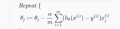# Machine learning logistic regression matlab code help

#### Saviour Muscat

Joined Sep 19, 2014
180
Hello helpers,

I am trying to do a logistic regression matlab code but with no success. Kindly could you indicate any mistakes did please.

Thankyou

#### Attachments

• 187.3 KB Views: 3
• 1.1 KB Views: 1

#### Papabravo

Joined Feb 24, 2006
20,397
I've done a lot of Math, but I have to admit that I've never heard of the concept. Can you offer a bit of explanation? Maybe I know it by a different name.

#### Saviour Muscat

Joined Sep 19, 2014
180
In my words Logistic regression is classification algorithm that makes a boundary(line) between two sets of data which can be for example pass or fail. Its activation function is the sigmoid function when the input is fed in the sigmoid its gives almost for all values one or zero. The sigmoid is function isFor my case z is a straight line z=intercept+gradient*datapoint(y=c+mx)
where the gradient decent formula isis similar to linear regression

In my code I tried to find the intercept and the gradient of the boundary line by gradient decent but something in my code is not working as should be. Please could you give me some hints to discover my mistakes please.

Thank you

#### Papabravo

Joined Feb 24, 2006
20,397
I don't think you need to go to that level of difficulty. You know the point (0, 0.5) is on the curve. The slope of an approximating line (not a tangent line) will be given by the derivative of S(z) as z approaches zero from either the left or the right. Once you have the slope you can write the equation of a line with the point slope formula and determine the y-intercept. Can you compute the derivative of S(z) as z approaches 0?

It also looks like an inflection point where the first derivative has a maximum which means the second derivative should have a zero.